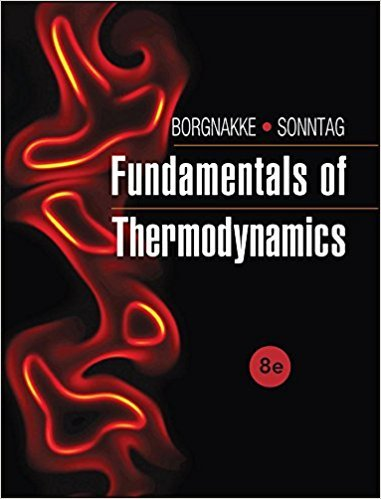×
Log in to StudySoup
Get Full Access to Fundamentals Of Thermodynamcs - 8 Edition - Chapter 5 - Problem 3cgp
Join StudySoup for FREE
Get Full Access to Fundamentals Of Thermodynamcs - 8 Edition - Chapter 5 - Problem 3cgp

Already have an account? Login here
×
Reset your password

# Suppose we forget the model for heat transfer, Q = CA ?T;ISBN: 9781118131992 58

## Solution for problem 3CGP Chapter 5

Fundamentals of Thermodynamcs | 8th Edition

• Textbook Solutions
• 2901 Step-by-step solutions solved by professors and subject experts
• Get 24/7 help from StudySoup virtual teaching assistantsFundamentals of Thermodynamcs | 8th Edition

4 5 1 363 Reviews
18
3
Problem 3CGP

Problem 3CGP

Suppose we forget the model for heat transfer, Q = CA ΔT; canwe drawsome information about the direction of Q from the second law?

Step-by-Step Solution:

Solution 3CGP

Step 1 of 3

The objective here is to evaluate if we can find out the direction of heat transfer from the second law if an equation for a heat transfer model is known.

Step 2 of 3

Step 3 of 3

##### ISBN: 9781118131992

Unlock Textbook Solution

Enter your email below to unlock your verified solution to:

Suppose we forget the model for heat transfer, Q = CA ?T;